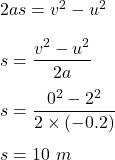## A girl rolls a basketball across a basketball court. The ball slowly decelerates at a rate of -0.20m/s^2. If the initial velocity was 2.0m/s

Question

A girl rolls a basketball across a basketball court. The ball slowly decelerates at a rate of -0.20m/s^2. If the initial velocity was 2.0m/s and the ball rolled to a stop at 5.0sec after. 12:00, at what time did she start the ball rolling?​

in progress 0
6 months 2021-07-19T00:53:18+00:00 1 Answers 63 views 0

## Answers ( )

1. By equation of motion :Now, it is given that it stops after 5 seconds of motion and time at that point is 12:00.

So, time when it started is 11 : 59 : 55.

Therefore, distance travelled is 10 m.

Hence, this is the required solution.